# Microsoft Access Regression Calculations with Total Access Statistics

Microsoft Access does not offer regression analysis, but writing your own regression calculations which involves matrix inversions is quite complicated and time consuming.

Fortunately, Total Access Statistics can generate regressions on your Microsoft Access tables and queries. All results are in MS Access tables that you can use on your forms, reports, and VBA code.

## Introduction to Regressions

A regression calculates the least squares (best fit) line or curve to determine an equation which relates the Y dependent variable to the X independent variable(s).

## Regression Feature in Total Access Statistics

Total Access Statistics makes it very easy to calculate the regressions in MS Access:

• Analyze the data from Microsoft Access tables and queries (including linked data)
• An unlimited number of records may be calculated in one pass.
• Optionally specify GROUP BY fields so you can perform regressions for each set of grouped records in one pass
• Optionally specify a weighting field
• Optionally specify a value or range of values to ignore

All the options can be selected interactively through the add-in Wizard interface. VBA developers can run the analysis through a programmatic VBA function call.

## Selecting Fields for Regressions Analysis

Field selection is very simple:

• One numeric field must be designated the Y field
• At least one X field must be assigned
• Group and Weight fields are optional

A separate calculation is made for each group of records (combination of unique Group fields values). A separate record is created for each group and X field.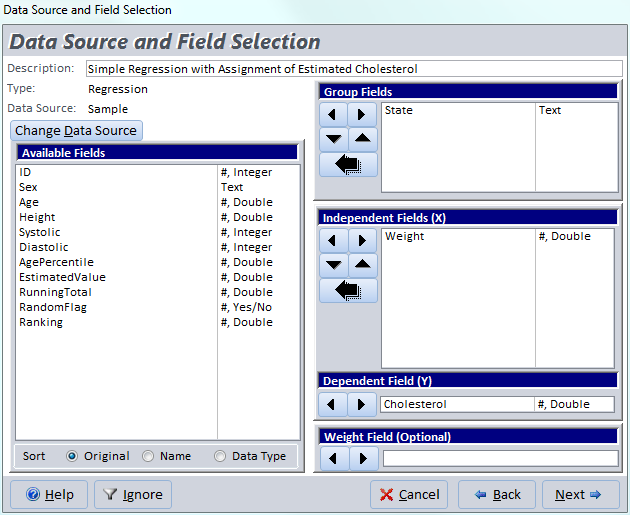Regression Field Selection for Cholesterol versus Weight for each State

After you select your fields and press the [Next] button, the Regression options appear.

## Regression Options in Total Access Statistics

Choose among three regression types and two calculation options:Total Access Statistics supports the generation of Simple, Multiple, and Polynomial regressions:

### Simple Linear Regression

A linear (simple) regression is calculated between the Y field and each X field using this simple equation: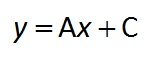where A and C are the constants that are solved. “A” is the slope of the line, “C” is the y-intercept. One equation (record) is created for each set of group fields and X field.

### Multiple Regression

A multiple regression of the linear combination of X fields to the Y field is calculated. All X fields are assumed to be independent variables. At least two X fields must be assigned; otherwise you should use simple regression. Here's the multiple regression equation: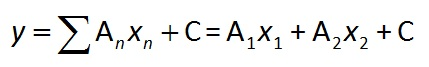where xi is the ith X field, and Ai and C are constants. Any record with a blank X field or Y field is ignored. One equation (record) is created for each group of check fields.

### Polynomial Regression

A polynomial regression between each X field and Y field is calculated based on this equation:where N is the polynomial order, and Ai and C are constants. The polynomial order (up to 9) determines the maximum power (exponent) of X in the equation. Any record with a blank X or Y field is ignored. One equation (record) is created for each group of check fields and X field.

### Calculate Y-Intercept

This option controls whether the y-intercept (C constant) is calculated or set to zero (through the origin). If this option is checked, the y-intercept is calculated.

### Calculate Regression ANOVA

If this option is selected, each regression’s ANOVA values are calculated. The field names are described later.

## Result Options

You can optional choose to create a residual table and assign the estimated Y value to a field in your data source:### Create Residual Table

If this option is selected, a separate Residual table is created. The residual table contains one record for each data point used in the regression calculation along with residual information. The Residual is the difference between the actual Y and the regression’s estimated Y value.

### Assign Estimated Y to Field

The value of Y based on the regression equation can be inserted directly into a field on each record. This feature is available when only one regression equation is being created for each record. This is always the case for a multiple regression. For simple and polynomial regressions, this exists if only one Independent (X) field is selected.

Simply specify the field where you’d like the result to be placed. The field should be a double and the data source must of course be updateable.

## Regression Output Tables

The following examples are different types of regressions for comparing X fields [Age] and [Weight] against Y Field [Cholesterol], grouped by [State] (not all fields shown).

### Simple Regression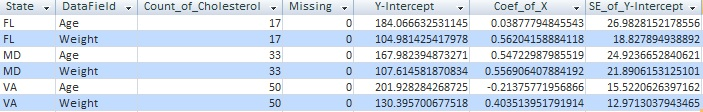### Multiple Regression### Polynomial Regression (Order 3)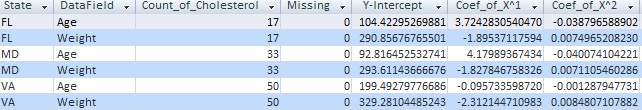## Regression Output Fields

Regardless of which options are selected, the output table for Regression always includes these fields:

Field Name Description
Group Fields Group fields selected (if any)
DataField Field name identifying the data in the record
Count of Y Field Number of records in the group, where Y Field is the name of the Y Field
Missing Records with missing values
Multiple R Square root of R-Square
R-Square R-Square measures the proportion of variation in Y that is explained by the regression. R-Square is between 0 and 1 (1 is a perfect fit).
StdError_of_Estimate Standard error of the Y estimate (regression) is the standard deviation of the residuals
Y-Intercept Y-Intercept value, if this option is selected
Coef_of_X Coefficient(s) of the regression equation

The regression coefficient fields vary depending on the type of regression selected:

• Simple Regressions: one field for the X coefficient.
• Multiple Regression: one field for each X field: Coef_of_X1, Coef_of_X2, Coef_of_X3, etc.
• Polynomial Regressions: one field for each polynomial order: Coef_of_X^1, Coef_of_X^2, Coef_of_X^3, etc.

## Coefficient Analysis Fields

In addition to the Y-Intercept and equation coefficients, four other fields are calculated for each coefficient to determine the accuracy and relative importance of each coefficient. The four fields are explained below with the field names in brackets:

### Standard Error of Coefficients [SE_of_X]

Standard Error of Coefficients is a measure of each coefficient’s variability. Although the coefficient is calculated, the sample data is assumed to contain variability. The population coefficient is the sample coefficient plus or minus the standard error.

### Beta Value of Coefficients [Beta_of_X]

Beta Value of Coefficients (also known as standardized regression coefficients) shows the relative importance of each coefficient in the regression equation. For multiple and polynomial regressions, it can be misleading to examine only the unstandardized coefficients for importance. A large coefficient may have little impact if its variable does not vary much. The Beta removes this effect. Beta is not calculated for the Y-Intercept.

### t-Value of Coefficients [t-Value_of_X]

The t-Value of the coefficient is used to determine (via t-Test) the probability that the coefficient value is significantly different from zero. t-Value is the ratio of the Coefficient to its Standard Error.

### Probability of Coefficients [Prob_of_X]

Probability of coefficients determines the probability that the coefficient is zero based on its t Value.

## Regression ANOVA Fields

The regression ANOVA indicates how much of the data variance is due to the regression, and how much is not (residual). If the regression has a poor “fit” (large residual), the F-Value is small and the probability that all regression coefficients are zero (the null hypothesis) approaches 1. These are the ANOVA fields:

Field Name Symbol Description
Regression_DF DF Regression degrees of freedom. Number of independent variables.
Residual_DF DF2 Residual degrees of freedom = N - DF1 - 1 (where N is the number of data points)
Total_DF DFT Total degrees of freedom = N – 1
Regression_SS SS1 Regression (estimated-average) sum of squares
Residual_SS SS2 Residual (actual-estimated) sum of squares
Total_SS SST Total sum of squares = SS1 + SS2
Regression_MS MS1 Regression mean squares = SS1 / DF1
Residual_MS MS2 Residual mean squares = SS2 / DF2
F-Value F Ratio of mean squares = MS1 / MS2
Prob P Probability (0-1) that all coefficients = 0

## Residual Table

If the Residual table option is selected, a second table is created: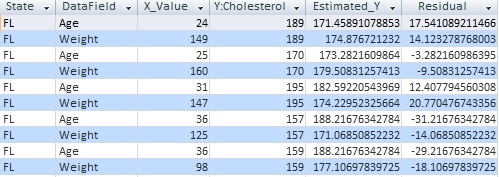This table contains all data (X and Y) used in the regression as well as the estimated Y value and the residual. A record is created for every data point. Data that is not used in the regression (blank values) or groups for which a regression could not be calculated are not included in the Residual table.

Field Name Description
Group Fields Group fields selected (if any)
DataField Field name identifying the data in the record
X Value Value of the X Field
Y Field Actual Y value from the data source
Estimated Y Estimated Y value based on the regression equation and the calculated coefficients
Residual Difference between the actual and estimated Y values

From the residual table, you can create a graph that plots the individual data points and a line through the estimated Y values to see the regression equation.

## Interactive Wizard and VBA Programmatic Interfaces

Total Access Statistics lets you interactively generate your calculations through its wizard interface to easily select the data source, fields, and analysis without programming. After you specify your selections, they are automatically saved as a scenario in your database so you can run them again in the future when your data changes.

A VBA statistics function is also available so you can generate these results from code. You can easily run any saved scenario by calling a single procedure in the Total Access Statistics VBA library. The library includes a royalty-free runtime license so you can include it with your Access database and distribute it to non-Total Access Statistics owners.

Call the function from a button's OnClick event or other process, and the results are generated for you. You can even hide the built-in user interface so your users don't even know Total Access Statistics is running. They'll be amazed with your statistical analysis capabilities!

## Additional Details of Calculations Available in Total Access Statistics

Here are some additional resources and details about some of the data analysis you can perform on your Microsoft Access data with Total Access Statistics:

## Total Access Statistics

### Microsoft Access 2016 and 2013 Versions are shipping!

Version 14.1 for
Microsoft Access 2010

Version X.9 for
Microsoft Access 2007 and 2003

Version X.8 for
Microsoft Access 2002, 2000, and 97

### Reviews

"Total Access Statistics performs a wide range of data analysis right inside Access. The product includes a Statistics Wizard to perform parametric, group, non-parametric, record analysis and more. The results are placed in Access tables that you can integrate into your forms and reports."

Alison Balter, Author

More Awards Reviews and References in Scientific Papers

### Statistics InfoPDF Factsheet